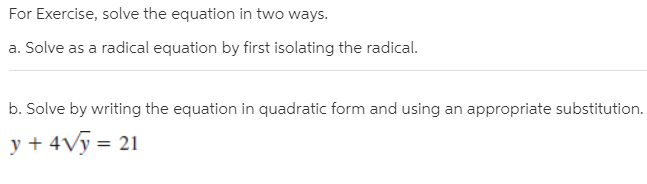# For Exercise, solve the equation in two ways. a. Solve as a radical equation by first isolating the radical. b. Solve by writing the equation in quadratic form and using an appropriate substitution. y + 4Vy = 21

Questionhelp_outlineImage TranscriptioncloseFor Exercise, solve the equation in two ways. a. Solve as a radical equation by first isolating the radical. b. Solve by writing the equation in quadratic form and using an appropriate substitution. y + 4Vy = 21 fullscreen